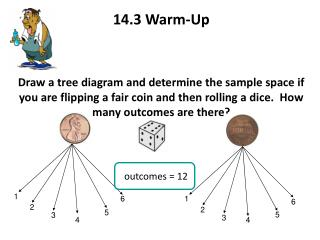# 14.3 Warm-Up - PowerPoint PPT PresentationDownload Presentation14.3 Warm-Up

Loading in 2 Seconds...

14.3 Warm-UpDownload Presentation## 14.3 Warm-Up

- - - - - - - - - - - - - - - - - - - - - - - - - - - E N D - - - - - - - - - - - - - - - - - - - - - - - - - - -
##### Presentation Transcript

1. 14.3 Warm-Up Draw a tree diagram and determine the sample space if you are flipping a fair coin and then rolling a dice. How many outcomes are there? outcomes = 12 1 6 1 6 2 2 5 5 3 3 4 4

2. Lesson 14.3 Probability:Determining Outcomes

3. Last lesson we learned 3 important things: 1. Probability is the chance that something will happen, 2. The result of each experiment is called an event or outcome 3. The set of all possible outcomes is called the Sample Space. We also discussed that probabilities are most commonly written as fractions. That is, the desired event (what we want to happen) over the total outcomes (the sample space). Can you guess what all of the probabilities would add up toof a given event? 1…One…Un…Uno….As in 100%

4. Let’s consider the marble example from the last lesson and take it one step further. Remember we were given 4 red marbles and 6 blue marbles. Determine the probability of drawing a blue marble. 6 blue marbles = 3 10 marbles total 5 What if we wanted to know the probability of picking a red marble (Not putting it back in the jar) and then picking a blue marble? 4 red marbles = 2 10 marbles total 5 6 blue marbles = 2 9 marbles total 3

5. Now let’s try a coin problem. Say you have three fair coins. Let’s find the following probabilities: • You get exactly one tail • You get at least one head • You get no tails Take a minute and write out the sample space for this problem, often times the sample space helps you determine your options. { HHH, HHT, HTH, THH, HTT, THT, TTH, TTT } • You get exactly one tail: 3/8 • You get at least one head (This means 1 or more!): 7/8 • You get no tails: 1/8

6. Sum of dice

7. Find the following probabilities if you roll 2 dice: • The sum of the roll is less than 7 • The sum of the points is exactly 10 • The sum of the roll is a prime number • The sum of the roll is divisible by 3 Now how many different pairs of numbers add up to less than 7? 1 1, 1 2, 1 3, 1 4, 1 5 2 1, 2 2, 2 3, 2 4, 3 1, 3 2, 3 3, 4 1, 4 2, 5 1 15 36 5 12 = How many different pairs of numbers add up to exactly 10? 3 36 1 12 4 6, 6 4, 5 5 =

8. A glass jar contains 6 red, 5 green, 8 blue and 3 yellow marbles. If a single marble is chosen at random from the jar, what is the probability of choosing a red marble? a green marble? a blue marble? a red or blue marble? P(red)  =  # of ways to choose red = 6 = 3 total # of marbles   22 11 P(green)  =  # of ways to choose green = 5 total # of marbles   22 P(blue)  =  # of ways to choose blue = 8 = 4 total # of marbles   22 11 P(red or blue)  =  # of ways to choose red + # of ways to choose blue total # of marbles   total # of marbles P(red or blue)  =  6 + 8 = 14 = 7 22 22 22 11

9. General Rule of Thumb: Often when working with probability, you will see key words such as “and” and “or” like we saw in the last marble problem. Something to keep in mind: “And” tells us to MULTIPLY the probabilities of each event “Or” tells us to ADD the probabilities of each event

10. Reflection: Explain what probability represents… Homework: 14.3 Worksheet